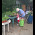April 12, 2015

Polynomial Long Division

Last summer during the TMC Nix the Tricks session Chris was really excited to figure out how to use the partial quotient method of long division for polynomials. I gave it as an option this year when refreshing my PreCalc students on the process but they preferred the standard algorithm of long division.

The week after, the calculus teacher said she'd been talking to an Algebra 2 teacher about long division and they'd figured out that you can both use partial quotients and you can start by dividing the constant rather than the variable. She gave this example:

So instead of starting with "x^3 divided by x" she started with "8 divided by 2." The claim being it's easier for kids to divide by two than to divide by x. The importance of building that fluency aside, it bothered me that we were going backward from the standard algorithm. At first it was just a niggling thought in the back of my mind, but after a while I realized the issue - this works great for polynomials that divide evenly, but what about remainders?

I posed the question to the other teachers and we tried some examples:

Left: Try the new method like you would with whole numbers - "5 divided by 2 is 2 (plus a remainder). That -1 never goes away and you get a remainder of 11x^2 - 1. But the remainder can't have a higher degree than the divisor. I protested so we continued dividing. We get there eventually.

Right: Try the new method but allow fractions - "5 divided by 2 is 5/2" The numbers get messy and we quit before finding our way to a remainder that has degree zero.

Right: The usual method of dividing by starting with the highest 'place value.' This goes much faster than our previous attempt (still on the left).

A few thoughts:
1) I would never have thought to start dividing with the smallest term! How cool that it works!
2) When I teach long division in PreCalc we graph first to find real roots and then divide to determine the imaginary roots - there's never a remainder. So does it matter that it's longer in that case?
3) A new teacher in my department said, "This is good but kids my quit if it doesn't work." I was a bad mentor and didn't stop to think. I immediately responded, "But that's what's so great about partial quotients! You don't have to back up if you didn't take out enough, you can just throw in another number/variable." Then I did think, and I realized he meant that kids would quit when they started introducing terms back in that they'd already eliminated (on the left the x term is zeroed out, then it comes back like a monster that can't be vanquished!). I apologized and asked if that's what he meant. Sometimes I'm so quick to defend the 'new methods' that I don't listen carefully to valid criticism.

1.This is really one of the most arduous and tedious things to do. It has a place but I find it's best role is a way for students to master all the details of polynomial manipulation.

1.Definitely. Which is why I have students graph first to find the nice roots. No guess and check with long division, it takes way too long!!

2.Isn't +10x -14x = -4x

1.The notation isn't ideal here because it was teachers playing. Should say (7x^2+10x-5)-(7x^2-14x). You're always subtracting the second line.

3.Starting from the constants is a great way to recover the Fibonacci sequence from the generator 1/(1-x-x^2).

1.